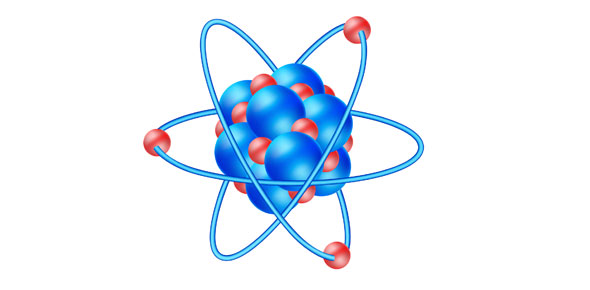# 1. [atomic Structure] Practice - Honors

6 Questions | Attempts: 185Settings• 1.
(a) Which of the following particles is the smallest?
• A.

Protons

• B.

Electrons

• C.

Neutrons

• D.

All of the above are the same size.

• 2.
(b) How many protons, electrons, and neutrons are in an average, neutrally-charged atom of Hydrogen?
• A.

1 proton, 1 electron, 1 neutron

• B.

1 proton, 0 electrons, 1 neutron

• C.

1 proton, 1 electron, 0 neutrons

• D.

1 proton, 2 electrons, 1 neutron

• 3.
(c) Which of the following elements has chemical and physical properties that are most similar to Magnesium?
• A.

Calcium

• B.

Sodium

• C.

Aluminum

• D.

Both Sodium and Aluminum

• 4.
(d) To which of the following families does Iodine belong?
• A.

Metalloids

• B.

Transition Metals

• C.

Noble Gases

• D.

Halogens

• 5.
(e) How many neutrons are in an atom of C-14?
• A.

6

• B.

12

• C.

8

• D.

14

• 6.
(f) Which of the following shows the correct calculation for determining the average atomic mass of copper based on the relative abundance data below?Cu-63 (69%)            Cu-65 (31%)
• A.

(63+65) / 2

• B.

(65-63) / 2

• C.

(65 / 2) + (63 / 2)

• D.

(63 x 0.69) + (65 x 0.31)

## Related TopicsBack to top
×

Wait!
Here's an interesting quiz for you.